# Non Verbal Reasoning - Grouping of Images

### Exercise :: Grouping of Images - Section 2

In each of the following questions, group the given figures into three classes using each figure only once.

1.

Group the given figures into three classes using each figure only once.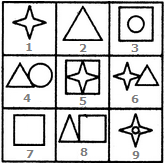A. 3,4,9 ; 5,7,8 ; 1,2,6 B. 1,5,6 ; 2,4,8 ; 3,7,9 C. 4,6,8 ; 3,5,7 ; 1,2,9 D. 1,2,7 ; 3,5,9 ; 4,6,8

Explanation:

1, 2, 7 are simple geometrical figures.

3, 5, 9 have one element placed inside a different element.

4, 6, 8 have two different elements attached to each other.

2.

Group the given figures into three classes using each figure only once.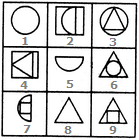A. 1,5,8 ; 3,4,7 ; 2,6,9 B. 1,3,6 ; 4,5,9 ; 2,7,8 C. 1,3,6 ; 2,5,7 ; 4,8,9 D. 6,7,8 ; 1,3,7 ; 2,4,9

Explanation:

1, 3, 6 contain one complete circle each.

2, 5, 7 contain a semi-circle each.

4, 8, 9 contain a triangle each.

3.

Group the given figures into three classes using each figure only once.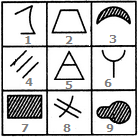A. 1,3,6 ; 4,5,8 ; 2,7,9 B. 2,3,9 ; 4,5,8 ; 1,6,7 C. 1,6,8 ; 3,7,9 ; 2,4,5 D. 3,8,9 ; 1,2,7 ; 4,5,6

Explanation:

1, 6, 8 are figures composed of straight as well as curved lines.

3, 7, 9 are closed figures shaded by oblique line segments.

2, 4, 5 are figures composed of straight lines only.

4.

Group the given figures into three classes using each figure only once.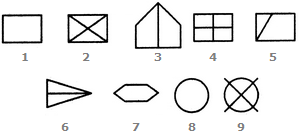A. 1,2,4 ; 3,5,6 ; 7,8,9 B. 1,7,8 ; 3,5,6 ; 2,4,9 C. 1,3,4 ; 2,8,9 ; 5,6,7 D. 1,7,8 ; 2,3,6 ; 4,5,9

Explanation:

1, 7, 8 are all undivided geometrical figures.

3, 5, 6 are geometrical figures divided into two parts.

2, 4, 9 are geometrical figures divided into four parts.

5.

Group the given figures into three classes using each figure only once.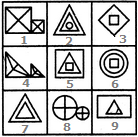A. 1,3,7 ; 2,4,6 ; 5,8,9 B. 1,4,6 ; 2,5,7 ; 3,8,9 C. 1,4,8 ; 2,5,6 ; 3,7,9 D. 1,4,8 ; 2,7,9 ; 3,5,6

Explanation:

1, 4, 8 contain similar elements (not equal in size) each divided into four parts and attached to each other.

2, 5, 6 contain three elements (two of which are similar) placed one inside the other.

3, 7, 9 contain one element inside the other, which may or may not be similar.